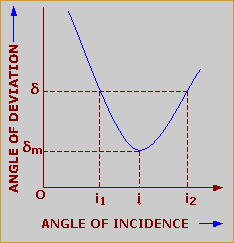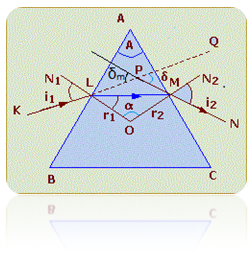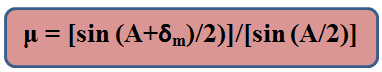×#### Thank you for registering.

One of our academic counsellors will contact you within 1 working day.

Click to Chat

1800-1023-196

+91-120-4616500

CART 0

• 0

MY CART (5)

Use Coupon: CART20 and get 20% off on all online Study Material

ITEM
DETAILS
MRP
DISCOUNT
FINAL PRICE
Total Price: Rs.

There are no items in this cart.
Continue Shopping

dreive an expression for the refractive index of the material of a prism in terms of angle of minimum deviation and angle of prism


2 years agoµ = sin i/sin r     (By Snell’s law)The refracted ray LM is incident on the face AC at the point M where N2MO is the normal and ∠r2 is the angle of incidence. Since the refraction now takes place from denser to rarer medium, therefore, the emergent ray MN such that ∠i2 is the angle of emergence.In the absence of the prism, the incident ray KL would have proceeded straight, but due to refraction through the prism, it changes its path along the direction PMN. Thus, ∠QPN gives the angle of deviation ‘δ’, i.e., the angle through which the incident ray gets deviated in passing through the prism. Thus,   δ = i1 – r1 + i2 -r2     ….... (1) δ = i1 + i2 – (r1 + r2 )Again, in quadrilateral ALOM,∠ALO + ∠AMO = 2rt∠s                [Since, ∠ALO = ∠AMO = 90º]So, ∠LAM +∠LOM = 2rt∠s           [Since, Sum of four ∠s of a quadrilateral = 4 rt∠s]    ….... (2)Also in ?LOM,∠r1 +∠r2 + ∠LOM = 2rt∠s         …... (3)Comparing (2) and (3), we get∠LAM = ∠r1 +∠r2 A = ∠r1 +∠r2 Using this value of ∠A, equation (1) becomes,δ = i1 + i2 - Aor i1 + i2 = A + δ                   …... (4)The angle of deviation of a ray of light in passing through a prism not only depends upon its material but also upon the angle of incidence. The above figure (2) shows the nature of variation of the angle of deviation with the angle of incidence. It is clear that an angle of deviation has the minimum value ‘δm’ for only one value of the angle of incidence. The minimum value of the angle of deviation when a ray of light passes through a prism is called the angle of minimum deviation.The figure (3) shows the prism ABC, placed in the minimum deviation position. If a plane mirror M is placed normally in the path of the emergent ray MN the ray will retrace its original path in the opposite direction NMLK so as to suffer the same minimum deviation dm.In the minimum deviation position, ∠i1 = ∠i2and so ∠r1 = ∠r2 = ∠r    (say)Obviously, ∠ALM = ∠LMA = 90º – ∠rThus, AL = LMand so LM l l BCHence, the ray which suffers minimum deviation possess symmetrically through the prism and is parallel to the base BC.Since for a prism,∠A = ∠r1 + ∠r2So, A = 2r      (Since, for the prism in minimum deviation position, ∠r1 = ∠r2 = ∠r)or r = A/2        …...(5)Again, i1 + i2 = A + δor i1 + i1 = A + δm         (Since, for the prism in minimum deviation position, i1 = i2 and δ = δm)2i1 = A + δmor i1 = (A + δm) / 2           …... (6)Now  µ = sin i1/sin r1 = sin i1/sin r µ = sin [(A + δm) / 2] / sin (A/2)               …... (7)2 years ago
							When the prism is at minimum deviation position “Angle of incidence$\angle{i}$ = Angle of emergence$\angle{e}$ i.e ($\angle{i}= \angle{e}$) and the angle of refractions at both the surfaces of the prism will be equal i.e$\angle{r_1}= \angle{r_2}$. From geometry we haved=i+e-A    ;${r_1}+{r_2}= A$but when the prism is in minimum deviation d=$D_m$ ,$D_m$=2i-A since$\angle{i}= \angle{e}$$\implies$2i = A+$D_m$$\implies$ i =$\frac{A+D_m}{2}$ – – – – – – –  –  – – – – (a)and 2r =A since$\angle{r_1}= \angle{r_2}= \angle{r}$$\implies$$r = \frac{A}{2}$ – – – – – – –  –  – – – – (b)But according to snell’s law$\mu= \frac{Sini}{Sinr}$substituting the values of  i and r in the above equation from equations (a) and (b) we get$\mu= \frac{sin\frac{(A+D_m)}{2}}{sin \frac{A}{2}}$

2 years ago
Think You Can Provide A Better Answer ?

## Other Related Questions on Organic Chemistry

View all Questions »### Course Features

• 731 Video Lectures
• Revision Notes
• Previous Year Papers
• Mind Map
• Study Planner
• NCERT Solutions
• Discussion Forum
• Test paper with Video Solution### Course Features

• 70 Video Lectures
• Revision Notes
• Test paper with Video Solution
• Mind Map
• Study Planner
• NCERT Solutions
• Discussion Forum
• Previous Year Exam Questions